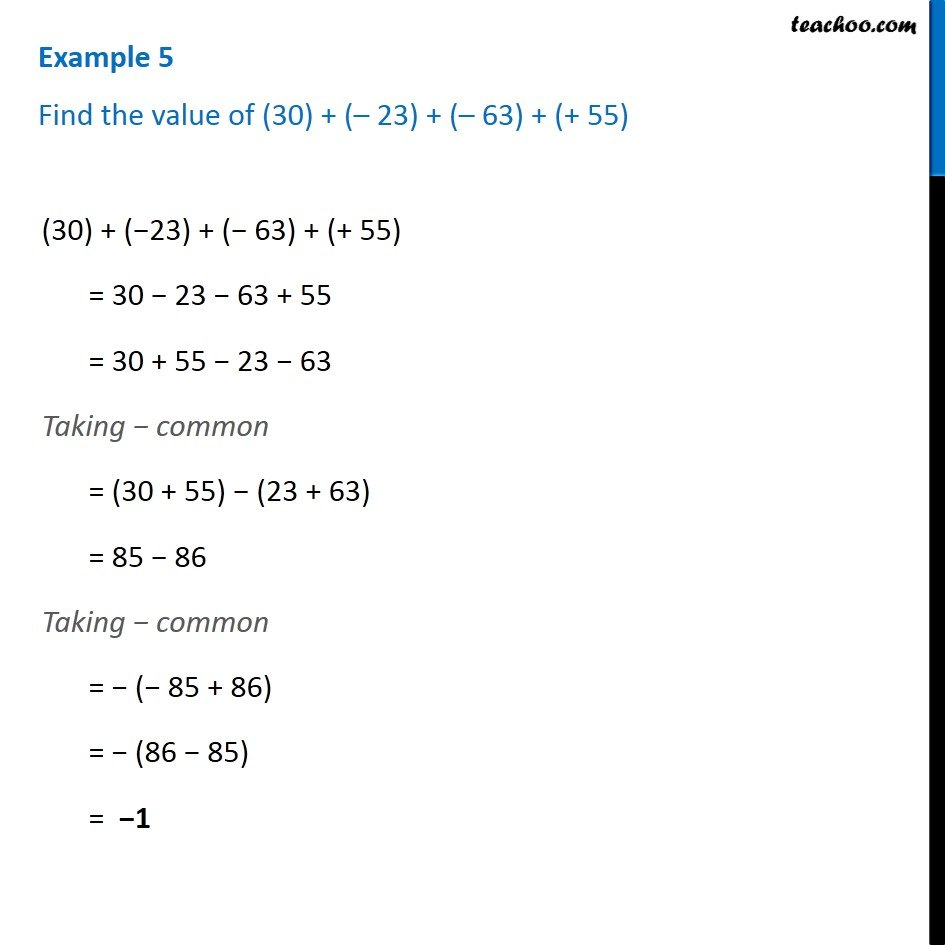1. Chapter 6 Class 6 Integers
2. Serial order wise
3. Examples

Transcript

Example 5 Find the value of (30) + ( 23) + ( 63) + (+ 55) (30) + ( 23) + ( 63) + (+ 55) = 30 23 63 + 55 = 30 + 55 23 63 Taking common = (30 + 55) (23 + 63) = 85 86 Taking common = ( 85 + 86) = (86 85) = 1